# Power function of a test

(diff) ← Older revision | Latest revision (diff) | Newer revision → (diff)
Jump to: navigation, search

A function characterizing the quality of a statistical test. Suppose that, based on a realizationof a random vectorwith values in a sampling space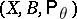,, it is necessary to test the hypothesis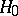according to which the probability distribution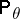ofbelongs to a subset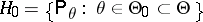, against the alternative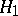according to which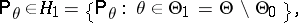and let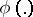be the critical function of the statistical test intended for testingagainst. Then(*)

is called the power function of the statistical test with critical function. It follows from (*) thatgives the probabilities with which the statistical test for testingagainstrejects the hypothesisifis subject to the law,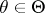.

In the theory of statistical hypothesis testing, founded by J. Neyman and E. Pearson, the problem of testing a compound hypothesisagainst a compound alternativeis formulated in terms of the power function of a test and consists of the construction of a test maximizing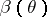, when, under the condition thatfor all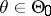, where(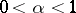) is called the significance level of the test — a given admissible probability of the error of rejectingwhen it is in fact true.